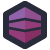Sandra’s ongoing battles continue. She currently works for Initrovent, and is doing her best to clean Karl’s dirty fingerprints off their event-planning codebase.

Now, as it turns out, Karl wasn’t their only developer. Another previous developer was a physicist who knew their way around APL and Fortran, then decided to follow the early 2000s money and became a self-taught web developer.

This Einstein decided to solve some problems, common problems, utility problems, the kind of things you might want to put in your central library and reuse in every project.

For example, do you hate comparisons? Does writing `if (\$x == \$y)…` make your skin crawl? Don’t you just wish you could write something like, `compareValues(\$x, \$y, '==')` instead?

Well, have I got news for you.

``````/**
* Compares two operands with an operator without using eval. Throws exception
* @param mixed  \$op1      Operand 1
* @param mixed  \$op2      Operand 2
* @param string \$operator Operator (==, !=, <=, >=)
* @return bool
*/
function compareValues(\$op1, \$op2, \$operator) {
switch (\$operator) {
case '==': return \$op1 == \$op2;
case '!=': return \$op1 != \$op2;
case '>=': return \$op1 >= \$op2;
case '<=': return \$op1 <= \$op2;
default:
throw new Exception('Operator is not handled: ' . \$operator);
}
}``````

Now, this particular snippet isn’t the worst thing on Earth, and it actually has a potential use- you might need to decide which operator to compare using, and store it in a variable.

What we really need is a way to route around PHP’s type system. This method does exactly that, but instead of being placed in a central library, it’s copy/pasted in five different places across multiple projects with slightly differing implementations.

``````    function compareValues(\$data1, \$operator, \$data2, \$type) {
if (!\$operator)
return false;
if (\$operator == '=')
\$operator = '==';
switch (\$type) {
case 'date':
if (is_array(\$data1)) {
\$data1 = \$data1['year'] . "-" .
\$data1['month'] . "-" . \$data1['day'];
}
if (is_array(\$data2)) {
\$data2 = \$data2['year'] . "-" .
\$data2['month'] . "-" . \$data2['day'];
}

\$data1 = strtotime(\$data1);
\$data2 = strtotime(\$data2);
if (empty(\$data1))
return 'empty';
if (empty(\$data2))
return 'empty';
return eval('return (' . \$data1 . \$operator . \$data2 . ');');
break;
case 'time':
if (empty(\$data1))
return 'empty';
if (empty(\$data2))
return 'empty';
\$data1 = (int) str_replace(':', '', \$data1); //removing ':'
\$data2 = (int) str_replace(':', '', \$data2);
return eval('return (' . \$data1 . \$operator . \$data2 . ');');
break;
case 'money':
if (empty(\$data2) || \$data2 == 'null') {
return 'empty';
}
if (\$this->options['Request_currency'] != \$this->options['Proposal_currency']) {
return false;
}
default:
if (empty(\$data1) && \$data1 !== 0)
return 'empty';
if (empty(\$data2) && \$data2 !== 0)
return 'epmty';
if (!is_numeric(\$data1))
\$data1 = '\'' . \$data1 . '\'';
if (is_string(\$data2))
\$data2 = '\'' . \$data2 . '\'';
return eval('return (' . \$data1 . \$operator . \$data2 . ');');
}
}``````

What a nice bit of defensive programming, ensuring that there’s a valid operator. And what a lovely pile of confusing code that may return `true`, `false` or FILE_NOT_FOUND `empty`. Or, sometimes, “epmty”. Bonus points for doing some of the comparisons using `eval`, thus allowing a code-injection attack. Einstein must have recognized this was bad, because some of the copy/pasted versions of this method instead called the first version of `compareValues` to avoid using `eval`.

But I know what you’re thinking. You’re thinking, “This is great stuff, but what good is having in PHP? This is 2018, and we’re writing operating systems in JavaScript now.” Well, don’t worry. This same method has a JavaScript version.

``````/**
* compares two values
*
*  @fieldId
*  @operator
*  @compareData
*
*/

function compareValues(data,operator,compareData,type,fieldId){
if(!operator) return;
if((data == "" || data == null) && (compareData != "" && compareData != null))
good = false; //when the data is im
else{
switch(type){
case 'date':
//changing the values to numeric strings
if(data.constructor == Array){
date1 = String(data)+String(data)+String(data);
}else{
date_arr = String(data).split("-");
if(date_arr.length == 2){ //swapping the order of the date-string (in case it's wrong)
date1 = String(date_arr+date_arr+date_arr);
}else
date1 = String(data).replace(/-/g,"");
}
if(compareData.constructor == Array){
date2 = String(compareData)+String(compareData)+String(compareData);
}else{
date_arr = String(compareData).split("-");
if(date_arr.length == 2){ //swapping the order of the date-string (in case it's wrong)
date2 = String(date_arr+date_arr+date_arr);
}else
date2 = String(compareData).replace(/-/g,"");
}
if(date1.length != 8 || date2.length != 8) //8 is the length of the number 'yyyymmdd'
return;
good = eval(date1+operator+date2);

break;
case 'money':
if (window.Request_currency != window.Proposal_currency)  {
good = false;
break;
}
default:
if(IsNumeric(data)){
good = eval(data+operator+compareData);
}else {
// Compare as strings
good = eval('\''+data+'\' '+operator+ ' \''+
compareData+'\'');
}
}
}
if(good){[Advertisement] Continuously monitor your servers for configuration changes, and report when there's configuration drift. Get started with Otter today!